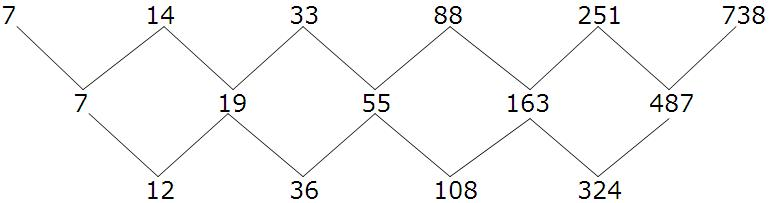Click to Chat

1800-1023-196

+91-120-4616500

CART 0

• 0

MY CART (5)

Use Coupon: CART20 and get 20% off on all online Study Material

ITEM
DETAILS
MRP
DISCOUNT
FINAL PRICE
Total Price: Rs.

There are no items in this cart.
Continue Shopping```Arithmeticogeometric Progression Solved ExamplesAs the name suggests, an arithmeticogeometric progression is obtained when the corresponding terms of a geometric and arithmetic progression are multiplied by each other.

Mathematically, it can be expressed as

xn = bnhn, where

xn is the nth term of the arithmeticogeometric progression

bn is the nth term of the arithmetic progression

hn is the nth term of the geometric progression

Illustration:

Find the sum of series 1. 2 + 2. 22 + 3. 23 +…+ 100. 2100.

Solution: Let us denote the given series by S.

Let S = 1.2 + 2.22 + 3.23 +…+ 100.2100                           …… (1)

⇒ 2S = 1.22 + 2.23 +…+ 99.2100 + 100.2101                      …… (2)

⇒ –S = 1.2 + 1.22 + 1.23 +…+ 1.2100 – 100.2101

⇒ –S = 1.2 (299–1/(2–1)) – 100.2101

⇒ S = –2100 + 2 + 100.2101 = 199.2100 + 2.

Illustration:

Let r = 1/2, consider nrn for increasing value of n and then find its value as n tends to infinity.

Solution: We first consider n (1/2)n for increasing values of n.

n = 1 we get 1. (1/2)1 = 1/2 = 0.5

n = 2 gives 2 × (1/2)2 = 1/2 = 0.5

n = 3 gives 3 × (1/2)3 = 0.375

n = 10 : 10 (1/2)10 = 0.00976, and so on

Thus we observe that as n → ∞
n rn → 0 for |r| < 1.

Illustration:

Evaluate 1 + 4/5 + 7/52 + 10/53 +…… to infinite terms.

Solution: We denote the given sequence by S.

Let S = 1 + 4/5 + 7/52 + 10/53 + ………

1/5 S = 1/5 + 4/52 + 7/53 ………

Subtracting

(1–1/5) S = 1 + 3/5 + 3/52 + 3/53 + ………

4/5 S = 1/ (1–3/5)                             (As it is infinite G.P.)

⇒ S =25/8

Refer the following video for more on arithmeticogeometric progression

Let t1, t2, t3, ……, tm–1, tm, tm+1, be a sequence so that

(i) tm+1/tm = tm/tm–1 ……… constant                             (r)

then tp = (t1)rp–1
(ii) tm+1/tm = tm/tm–1 = constant                                  (r)

then tp = constant 1 + (constant 2) × rp–1

(iii) If the difference of difference of terms are in G.P. then

tp = a + bp + crp–1, where r is the common ratio.

Illustration:

7. 14. 33. 88. 251. 738 …………

Solution: We can represent the given sequence in the form of a diagram as shownRemark: First we write down all the numbers in a straight line. Then we find out the difference between every pair of two terms. This is denoted in the second line. Again we find the difference between every pair of consecutive terms in the second line. Now we divide every successive term by the preceding term and see that the ratio in each case is 3.

324/108 = 108/36 = 36/12 = 3

Hence, using the formula listed above

∴      tp = a + bp + c 3p–1

p=1 so t1 = 7 = a + b + c

p=2 so t2 = 14 = a + 2b + 3c

p=3 so t3 = 33 = a + 3b + 9c

Solving, we get a = 3, b = 1, c = 3

⇒     tp = 3 + p + 3. (3p–1)
askIITians offers extensive study material which covers all the important topics of IIT JEE Mathematics. Topics like arithmetic progression, geometric progression and arithmeticogeometric progression have been covered along with numerous solved examples. It is important to have clear fundamentals in order to remain competitive in the JEE.

Related resources:

Look into the Previous Year Papers with Solutions to get a hint of the kinds of questions asked in the exam.

You can get the knowledge of Useful Books of Mathematics here.

To read more, Buy study materials of Sequences and Series comprising study notes, revision notes, video lectures, previous year solved questions etc. Also browse for more study materials on Mathematics here.
```### Course Features

• 731 Video Lectures
• Revision Notes
• Previous Year Papers
• Mind Map
• Study Planner
• NCERT Solutions
• Discussion Forum
• Test paper with Video Solution